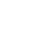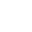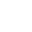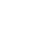﻿ DEPARTMENT OF AUDIO & VISUAL ARTS

# Mathematics for Audiovisual Technology

Teaching Staff: Panagopoulos Michail
Course Code: TEC414
Course Category: General Background
Course Type: Elective
Course Language: Greek
Delivery method: Lectures
Semester: 4th
ECTS: 7
Teaching Units: 4
Teaching Hours: 4
Teaching Structure:
Lectures26
Lab Lectures26
Literature Study and Analysis80
Practice and Preparation43
Course Total (ECTS: 7)175

Recquired / Recommended : THE104
Prerequisite to / Recommended to: (AUD623), (AVA745), VIS832

Short Description:

In the context of this course, basic knowledge required for understanding concepts concerning mostly issues of technological nature is given. The course is organised in four thematic units (analysis, algebra, analytic geometry and probabilities-statistics), in which the theoretical approach and presentation of the subjects and the respective mathematical concepts are combined with concrete examples of audiovisual technologies applications (e.g. development of algorithms for interactive audiovisual applications). Special emphasis is placed on the application of mathematical concepts through examples and drills related to sound and image technologies, using the mathematical package Matlab.

Objectives - Learning Outcomes:

The objective of the course is to provide to the students a general overview of the fondamental mathematics that are used in the audio and image processing together with the mathematics needed for 2d and 3d computer graphics.

The successful attendance of the course offers the ability to the corresponding students to:

• draw plots of functions and curves both in theory and in the computer
• know the derivatives (one or two variables) and calculate intergals
• understand analytic geometry and descrete mathematics
• write code in Octave (or MATLAB) that is applied for mathematical transforms

Syllabus:

1st Week

General introduction to the course. Basic elements of function plots in programming environment.

LAB:Introduction to Octave environment. Running simple programms for creating the plots of strait lines and parabolas

2nd Week

Functions: limits, continuous functions, derivatives. Basic functions (linear, logarithmic, exponential etc). Even – odd functions, invert function, composite functions.

LAB: Plots of cirlces in Cartesian and polar coordinates

3rd Week

Calculus. Derivatives – derivatives of basic functions. The derivative of the sum and product of functions. Local minimum and local maximum

LAB:

4th Week

Integrals: Introduction to integrals – antiderivative integral – definite integral. Integrals of basic functions. Trapezium method.

LAB: Plot of the transverse on specific point

5th Week

Integration with substitution – integration by parts. Calculation of the curve length

LAB: Run and analyse the trapezium methond on computer

6th Week

Multiple variable functions – partial derivatives.

LAB: Calculation of curve length

7th Week

Chain rule – examples. Vectors – internal product – external product

LAB: Spiral graphics – (2d and 3d)

8th Week

Vector analysis: Vector functions – 2 variable vector functions . Gradient of functions

LAB: Sphere plotting

9th Week

Vertical vector - transverse

LAB:Cone graphic (cylinder)

10th Week

Fourier tranform, Fourier sequence, Fourier integral

LAB: Descrite Fourier transform (FFT)

11th Week

Sequenses – Series – definitions, sequence limit – arithmetic and geometric series. Convergent series – divergent series

LAB: Solving 3x3 systems

12th Week

Combinatorics – Probabilities: ordering – permulation. Ordering with repeat. District probability

LAB: Plots of probability functions

13th Week

General overview – past exams exercises

Suggested Bibliography:

Wrede Robert C., Spiegel Murray R. (2015). Ανώτερα Μαθηματικά – Σειρά Schaum

B.S.Grewal (2014). Higher Engineering Mathematics. Khanna Publishers

Teaching Methods:

Teaching is taking place in the classroom where the basic theory is presentment together with provided examples. The lab part of the course includes practice with coding

Evaluation Methods:

The evaluation is a result of the final written exam.

Updated: 02-10-2018

 << < September 2022 > >> Mo Tu We Th Fr Sa Su 1 2 3 4 5 6 7 8 9 10 11 12 13 14 15 16 17 18 19 20 21 22 23 24 25 26 27 28 29 30
Today, Friday 30-09-2022
Ionian Contemporary Animation Festival ICONA 2022
Start: 24-08-2022 |End: 02-10-2022
[In Progress]
Tsirigoti Sq. 7, 49100 Corfu
Τel.: 26610 87860-1 - Fax: 26610 87866
e-mail: audiovisual@ionio.grText To SpeechText ReadabilityColor Contrast